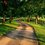# [Differential Geometry] What does an Isometry on Smooth Manifolds preserve?

We will look into $\varphi$, a diffeomorphic map, which is an invertible function that maps one differentiable manifold to another in a smooth way, where its inverse is also smooth. Now what are some things that can be preserved under these diffeomorphic maps? Let's look into what we call an isometry. If $\varphi: S \rightarrow \overline{S}$ is an isometry between two smooth manifolds $S$ and $\overline{S}$, then for all points $p \in S$ and all pairs of of tangent vectors $v$, $w$ in $T_p(S)$,

$\left< v , w \right>_p = \left _{\varphi(p)}.$

This means that the inner product of all tangent vectors at point $p$ in surface $S$ is preserved by the differential $d\varphi$ of the map. If the inner product is preserved, then the first fundamental form, hence the coefficients of the first fundamental form are also preserved by the isometry from the following:

$2I_p(v) = \left_p = \left_{\varphi(p)} = 2I_{\varphi(v)}(d\varphi(v)).$ $\therefore I_p(v) = I_{\varphi(v)}(d\varphi(v)).$

Therefore, the first fundamental form, $I_p(S)$ is also preserved under an isometry.

On the other hand, if we know that the first fundamental form is preserved under an isometry, then we can also conclude that the inner product of any pair of vectors on the tangent plane is preserved too, By polarisation, $2\left_p = I_p(v+w) - I_p(v) - I_p(w) = I_{d\varphi(p)}(v+w) - I_{d\varphi(p)}(v) - I_{d\varphi(p)}(w) = 2\left_{\varphi(p)}.$

$\therefore \left_p = \left_{\varphi(p)}.$Note by Bright Glow
3 years, 3 months ago

This discussion board is a place to discuss our Daily Challenges and the math and science related to those challenges. Explanations are more than just a solution — they should explain the steps and thinking strategies that you used to obtain the solution. Comments should further the discussion of math and science.

When posting on Brilliant:

• Use the emojis to react to an explanation, whether you're congratulating a job well done , or just really confused .
• Ask specific questions about the challenge or the steps in somebody's explanation. Well-posed questions can add a lot to the discussion, but posting "I don't understand!" doesn't help anyone.
• Try to contribute something new to the discussion, whether it is an extension, generalization or other idea related to the challenge.

MarkdownAppears as
*italics* or _italics_ italics
**bold** or __bold__ bold
- bulleted- list
• bulleted
• list
1. numbered2. list
1. numbered
2. list
Note: you must add a full line of space before and after lists for them to show up correctly
paragraph 1paragraph 2

paragraph 1

paragraph 2

[example link](https://brilliant.org)example link
> This is a quote
This is a quote
    # I indented these lines
# 4 spaces, and now they show
# up as a code block.

print "hello world"
# I indented these lines
# 4 spaces, and now they show
# up as a code block.

print "hello world"
MathAppears as
Remember to wrap math in $$ ... $$ or $ ... $ to ensure proper formatting.
2 \times 3 $2 \times 3$
2^{34} $2^{34}$
a_{i-1} $a_{i-1}$
\frac{2}{3} $\frac{2}{3}$
\sqrt{2} $\sqrt{2}$
\sum_{i=1}^3 $\sum_{i=1}^3$
\sin \theta $\sin \theta$
\boxed{123} $\boxed{123}$